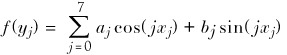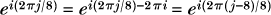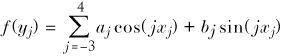»  Home »  Resources & support »  FAQs »  Discrete fast Fourier transform

## How can I calculate the Fourier coefficients of a discretely sampled function in Stata?

 Title Discrete fast Fourier transform Author May Boggess, StataCorp

For many of us, the last time we saw a Fourier series was in math class, where the goal was to decompose a function on [0,2π] into its frequency components by expressing it as a linear combination of {sin(nx), cos(nx), n=0... infinity}. The coefficients of this linear combination are called the Fourier coefficients.

In real life, we do not have the entire function at our disposal; we have just a sample, that is, a finite set of values of the function at particular points. If we sample 8 points, we cannot hope to determine an infinite number of coefficients. With 8 points, we will only be able to calculate 8 complex coefficients. Thus we usually try to sample as many points as we can. Fortunately, the fast Fourier transform is an algorithm for computing the coefficients that is, well, very fast (Monahan 2001, sec. 14.5).

You should to be aware that the FFT algorithm requires the number of sampled points to be a power of 2. If you do not, you can make more points by padding with zeros, but that introduces a minor complication (that we will discuss later), so it is easier to stick with 2m points.

The FFT algorithm for calculating the coefficients is well documented (Bloomfield 2000, sec. 5.7; Olver and Shakiban 2006). For 8 sampled points, we obtain 8 complex coefficients, that is, 8 pairs of real numbers. Using these coefficients, we can write down a function that is a sum of sines and cosines that passes through all the 8 sampled pointswhere (xj, yj) are the sampled points and aj, bj are the Fourier coefficients. The FFT algorithm requires that the x-values be equally spaced, so, for 8 points on [0,2π], we would have xj = 2π j/8, j=0...7. Becausewe can write the above sum using lower frequency components:### Simple examples

For our first example, let’s use y=sin(x) on [0,2π] with 8 sampled points (we are using a trivial example so that it will have nice Fourier coefficients):

 clear
set obs 8
gen x=(_n-1)*(2*_pi)/8
gen y=sin(x)
mata: h=st_data(.,"y")
mata: fft(h)
# delimit;
twoway scatter y x ||
function y=1/8*(4*sin(1*x)-4*sin( 7*x)), range(x) ||
function y=1/8*(4*sin(1*x)-4*sin(-1*x)), range(x) ||,
legend(cols(1) label(1 "sampled values")
label(2 "sum of high frequency components")
label(3 "sum of low frequency components"))
title("Reconstructed signal for y=sin(x) sampled at 8 points");
# delimit cr


The low-frequency components are often preferred. As the above example showed, the order of the coefficients Mata put into the matrix H is 0 though 7. To make it easy to use the low-frequency components, the Mata function ftunwrap() orders the coefficients in the low-frequency way: −3, −2, −1, 0, 1, 2, 3, 4. Let’s see this with another example:

 clear
set obs 8
gen x=(_n-1)*(2*_pi)/8
gen y=sin(x)+cos(2*x)
mata:
h=st_data(.,"y")
H=fft(h)
re=Re(H);im=Im(H)
re=ftunwrap(re);im=ftunwrap(im)
st_matrix("H",(re, im))
end
matrix list H
set obs 101
gen xhat=(_n-1)*2*_pi/100
# delimit;
gen yhat=(1/8)*(
H[1,1]*cos(-3*xhat)+H[1,2]*sin(-3*xhat)
+H[2,1]*cos(-2*xhat)+H[2,2]*sin(-2*xhat)
+H[3,1]*cos(-1*xhat)+H[3,2]*sin(-1*xhat)
+H[4,1]*cos(0*xhat) +H[4,2]*sin(0*xhat)
+H[5,1]*cos(1*xhat) +H[5,2]*sin(1*xhat)
+H[6,1]*cos(2*xhat) +H[6,2]*sin(2*xhat)
+H[7,1]*cos(3*xhat) +H[7,2]*sin(3*xhat)
+H[8,1]*cos(4*xhat) +H[8,2]*sin(4*xhat));
twoway scatter y x || line yhat xhat,
legend(label(1 "sampled points")
label(2 "reconstructed signal"))
title("Reconstructed signal for y=sin(x)+cos(2x)");
# delimit cr


Let’s go back to the minor complication we mentioned earlier: what happens if we do not have 2m sampled points? Suppose we have 100 points. In this case, we add 28 zeros on the end of the function using the Mata function ftpad() so that 128 points are “sampled”. That means Mata will have the wrong impression about what the x-values are for the sampled points: it will think we have 128 equally spaced points and the last 28 of them have zero y-value:

 clear
set obs 100
gen x=(_n-1)*(2*_pi)/100
gen y=x^2-2*x+1
mata:
h=st_data(.,"y")
re=Re(H);im=Im(H)
re=ftunwrap(re);im=ftunwrap(im)
st_matrix("H",(re, im))
end
local j=0
gen yhat=0
forvalues i=-63(1)64{
local j=j'+1
quietly replace yhat=yhat+H[j',1]*cos(i'*x) /*
*/                  +H[j',2]*sin(i'*x)
}
replace yhat=(1/128)*yhat
#delimit;
twoway scatter y x, msize(vsmall) || line yhat x,
legend(label(1 "sampled points")
label(2 "incorrect reconstructed signal"))
title("Incorrect reconstructed signal for y=x^2-2x+1")
subtitle("sampled at 100 points");
#delimit cr


Well, we certainly have not got that right. When we get the coefficients back from Mata, we should rescale and cut off the last piece of the function to get the original function back again:

 clear
set obs 100
gen x=(_n-1)*(2*_pi)/100
gen y=x^2-2*x+1
mata:
h=st_data(.,"y")
re=Re(H);im=Im(H)
re=ftunwrap(re);im=ftunwrap(im)
st_matrix("H",(re, im))
end
gen realx=(_n-1)*(2*_pi)/128 in 1/100
local j=0
gen yhat=0
quietly forvalues i=-63(1)64{
local j=j'+1
replace yhat=yhat +H[j',1]*cos(i'*realx) /*
*/               +H[j',2]*sin(i'*realx)
}
replace yhat=(1/128)*yhat
#delimit;
twoway scatter y x, msize(small) ms(Oh)|| line yhat x,
legend(label(1 "sampled points")
label(2 "correct reconstructed signal"))
title("Correct reconstructed signal for y=x^2-2x+1")
subtitle("sampled at 100 points");
#delimit cr


### The inverse Fourier transform

If we have sampled many points, we do not need to reconstruct the function using sin and cos (because we can get a good graph using just the points we have). In this case, we can use the Mata function invfft() to transform back from the coefficients to the y-values of the sampled function:

 clear
set obs 128
gen x=(_n-1)*(2*_pi)/128
gen y=sin(x)+cos(2*x)
gen yhat=.
mata:
h_in=st_data(.,"y")
H=fft(h_in)
h_out=invfft(H)
h_out
st_store((1,128),"yhat",h_out)
end
twoway scatter y x || line yhat x,  /*
*/ legend(label(1 "sampled points") /*
*/   label(2 "reconstructed signal")) /*
*/ title("Using FFT and invFFT on y=sin(x)+cos(2x)")


### Applications: filters and data compression

There are two common applications of Fourier series. The first is to filter out high-frequency noise in a sound signal (Olver and Shakiban 2006, 285; Bloomfield 2000, sec. 5.7). We can reduce the noise in a signal by taking its Fourier decomposition and discarding the high frequency components, that is, setting the high frequency coefficients to zero (note if we had sampled 8 points, the highest frequencies would be 3 and 4, not 6 and 7) (Boggess and Narcowich 2001, 139):

 clear
set obs 256
gen t=(_n-1)*(2*_pi)/256
gen y=exp(-(cos(t))^2)*(sin(2*t)+2*cos(4*t)+0.4*sin(t)*sin(50*t))
gen yhat=.
gen coef=.
mata:
h_in=st_data(.,"y")
H=fft(h_in)
H[(6::249),.]=J(244,1,0)
h_out=invfft(H)
h_out=Re(h_out)
st_store((1,256),"yhat",h_out)
end
twoway line yhat y t, /*
*/ legend(order(2 1) label(2 "sampled points") /*
*/   label(1 "filtered signal")) /*
*/ title("Filtered y=exp(-cos^2(t))(sin(2t)+2cos(4t)+0.4sin(t)sin(50t))")


A second common application is data compression (Boggess and Narcowich 2001, 139). In this case, we discard the coefficients with least absolute value:

 clear
set obs 256
gen t=(_n-1)*(2*_pi)/256
gen y=exp(-t^2/10)*(sin(2*t)+2*cos(4*t)+0.4*sin(t)*sin(10*t))
gen yhat=.
mata:
h_in=st_data(.,"y")
H=fft(h_in)
abs=abs(H)
sorted=uniqrows(abs)
sorted[205,1]
sorted[206,1]
for (i=1; i<=256; i++){
if (abs(H[i,1])<sorted[205,1]){
H[i,1] = 0
}
}
H
h_out=invfft(H); h_out=Re(h_out)
st_store((1,256),"yhat",h_out)
end
twoway line yhat y t, /*
*/ legend(order(2 1) label(2 "sampled points") /*
*/   label(1 "80% compressed signal")) /*
*/ title("80% compression") /*
*/ subtitle("y=exp((-t^2/10))(sin(2t)+2cos(4t)+0.4sin(t)sin(10t))")


### References

Bloomfield, P. 2000.
Fourier Analysis of Time Series. New York: Wiley.
Boggess, A., and F. Narcowich. 2001.
A First Course in Wavelets with Fourier Analysis. Upper Saddle River, NJ: Prentice Hall.
Monahan, J. 2001.
Numerical Methods of Statistics. Cambridge: Cambridge University Press.
Olver, P. and C. Shakiban. 2006.
Applied Linear Algebra. Upper Saddle River, NJ: Prentice Hall.